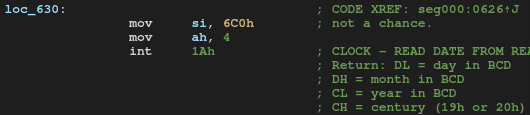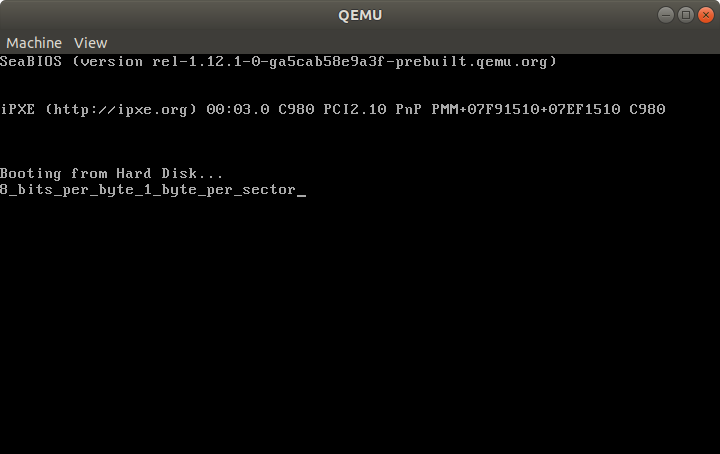# Codegate CTF 2020 Preliminary Writeup

I played Codegate CTF 2020 in shibad0gs. I was busy for another upcoming event and couldn't work on it full time but I solved some challenges and we reached 30th place.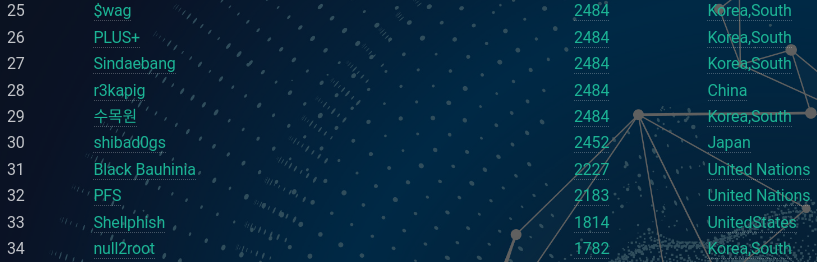As the challenge doesn't have category, I randomly picked up tasks.

# [333pts] SimpleMachine

We're given a stripped ELF possibly written in C++, and a file named target. It's a simpe VM and the target code accepts user input and validates if it's correct. Every instruction is 8-byte length and the first byte determines the instruction. This is the switch statement: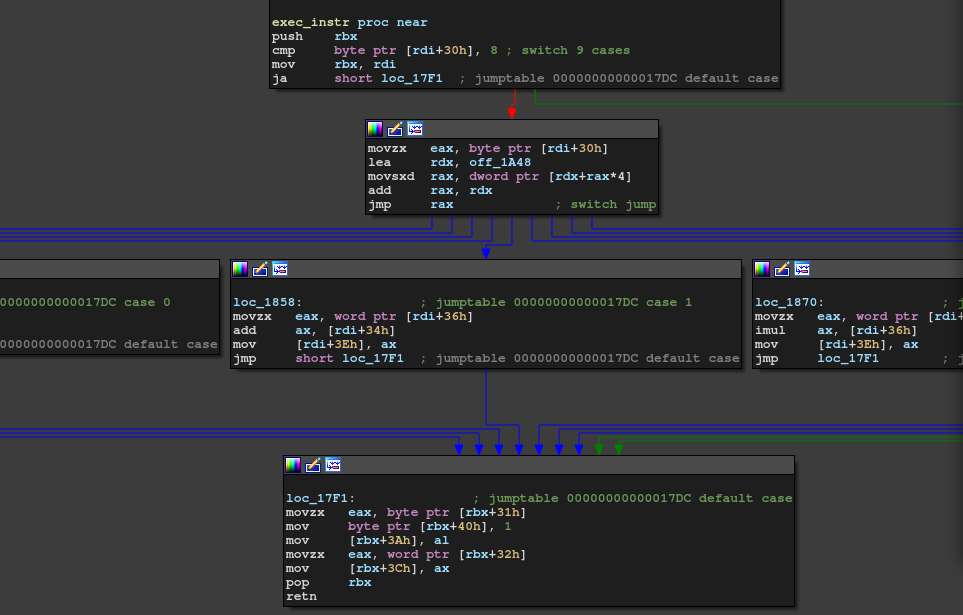It has only 8 instructions: mov, add, mul, xor, cmp, jnz, read, write. I wrote gdb script which forces it to trace the correct path and dumped the traces.

# gdb -n -q -x solve.py ./simple_machine
import gdb
import re

def get_arg():
v = int(re.findall("\t0x([0-9a-f]{8})", gdb.execute("x/1xw $rdi + 0x34", to_string=True)), 16) return v & 0xffff, v >> 16 gdb.execute("break *0x00005555555558c0") gdb.execute("break *0x00005555555558a0") gdb.execute("break *0x0000555555555888") gdb.execute("break *0x0000555555555870") gdb.execute("break *0x0000555555555858") gdb.execute("break *0x0000555555555848") gdb.execute("break *0x0000555555555830") gdb.execute("break *0x0000555555555810") gdb.execute("break *0x00005555555557e0") gdb.execute("run ./target < input") flag = '' log = '' skip = False while True: rip = int(re.findall("0x([0-9a-f]+)", gdb.execute("i r$rip", to_string=True)), 16)
if rip == 0x00005555555557e0:
arg1, arg2 = get_arg()
log += "[+] read 0x{:04x}, 0x{:04x}\n".format(arg1, arg2)
elif rip == 0x0000555555555848:
arg1, arg2 = get_arg()
log += "[+] mov 0x{:04x}, 0x{:04x}\n".format(arg1, arg2)
elif rip == 0x0000555555555858:
arg1, arg2 = get_arg()
log += "[+] add 0x{:04x}, 0x{:04x}\n".format(arg1, arg2)
#w = 0x10000 - arg2
#gdb.execute("set {{short}}*{{$rdi + 0x34}} = {}".format(w)) #flag += hex(w)[2:].decode("hex")[::-1] #print("[!] flag = {}".format(flag)) elif rip == 0x0000555555555870: arg1, arg2 = get_arg() log += "[+] mul 0x{:04x}, 0x{:04x}\n".format(arg1, arg2) elif rip == 0x0000555555555888: arg1, arg2 = get_arg() log += "[+] xor 0x{:04x}, 0x{:04x}\n".format(arg1, arg2) elif rip == 0x00005555555558a0: arg1, arg2 = get_arg() if arg2 == 0xc: skip = True else: skip = False log += "[+] cmp 0x{:04x}, 0x{:04x}\n".format(arg1, arg2) elif rip == 0x00005555555558c0: arg1, arg2 = get_arg() log += "[+] jnz 0x{:04x}, 0x{:04x}\n".format(arg1, arg2) if not skip: gdb.execute("set {short}*{$rdi + 0x34} = 0")
elif rip == 0x0000555555555810:
with open("trace.txt", "w") as f:
f.write(log)
break
gdb.execute("continue")


This dumps something like this:

[+] read 0x4000, 0x0024
[+] cmp 0x0000, 0x0000
[+] jnz 0x0000, 0x01a0
[+] cmp 0x0000, 0x0000
[+] jnz 0x0000, 0x01a0
[+] cmp 0x0000, 0x0000
[+] jnz 0x0000, 0x01a0
...

Just read the trace and wrote the solver.

from ptrlib import *

flag = b''
flag += p16(0x10000 - 0xb0bd)
flag += p16(0x10000 - 0xbabc)
flag += p16(0x10000 - 0xbeb9)
flag += p16(0x10000 - 0xbaac)
flag += p16(0x10000 - 0xcfce)
flag += p16(0x10000 - 0xcfce)
keyList = (
(0x63f7, 0xf974),
(0xa419, 0x2b9d),
(0xec2b, 0x4caf),
(0x347d, 0xbee1),
(0x5c87, 0xfc0d),
(0xe589, 0x6e48),
(0x2e9b, 0xe03c),
(0x94f7, 0x1979),
(0xbd19, 0x36d6),
(0xc72b, 0x40e8),
(0x497d, 0xcbf7),
)
for key in keyList:
for x in range(0x10000):
if (x ^ key) + key == 0x10000:
break
flag += p16(x)
print(flag)


That's it.

CODEGATE2020{ezpz_but_1t_1s_pr3t3xt}

# [702pts] malicious

We're given a 32-bit PE. In the first phase, the program initializes a buffer, which turned out to be decryption key later. As I didn't know it's important, I skipped this phase at first. In sub_403db11 has a code decryption process.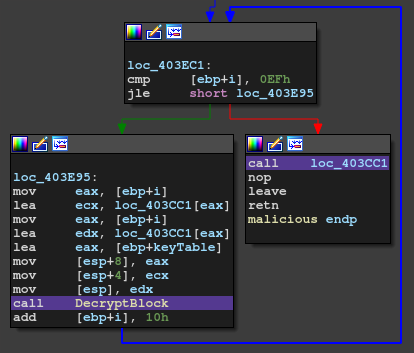By googling the magic numbers, I found the used cipher was Camellia. So, we need to get the key but it's the one the program initialized in the first phase. Since a remote server feeds the latter part of the key and it's down, we can't get the key. However, there's a check for the key.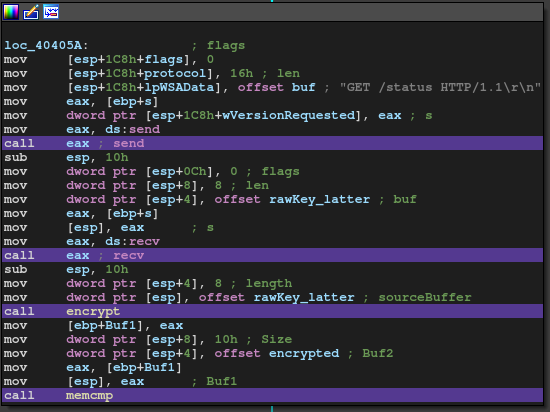I had been stuck here as sub_4039be was too complex to analyse. After a while, @akym found it's just a MD5. Also he dumped the decrypted PE :-)

Now we're in the second stage. In this stage, the program decrypts and writes bootloader to PhysicalDrive1000. I decrypted and dumped the bootloader.

#include <openssl/camellia.h>
#include <stdio.h>
#include <stdlib.h>
#include <string.h>

unsigned char code[0x4400];
int main() {
FILE *fp = fopen("hoge.exe", "rb");
fseek(fp, 0x4e40, SEEK_SET);
fclose(fp);

CAMELLIA_KEY keyTable = {0};
Camellia_set_key(rawKey, 0x80, &keyTable);
for(int i = 0; i < 0x4400; i += 0x10) {
Camellia_decrypt(&code[i], &code[i], &keyTable);
}

fwrite(code, 1, 0x4400, fp);
fclose(fp);
}


Okay, now we're in the third stage.

\$ file bootloader
bootloader: DOS/MBR boot sector MS-MBR

Another unpacker......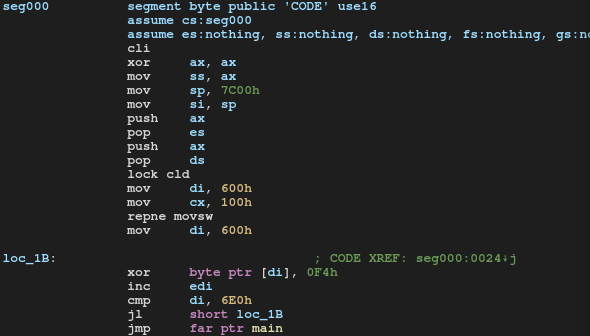Decode.

with open("bootloader", "rb") as f:

for c in code:
output += bytes([c ^ 0xf4])